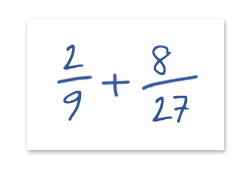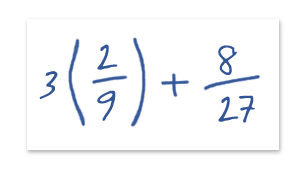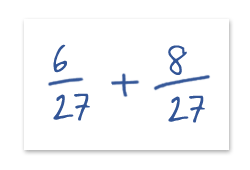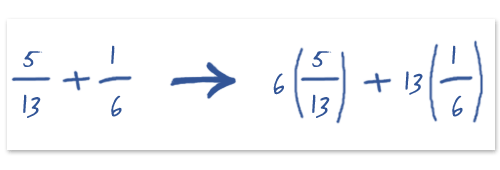# Category Archives: Fractions

## Classic Question 1: Add these two fractions and express the answer as a fraction

Adding fractions is something which doesn’t come up very often in numerical reasoning tests, however being confident in knowing how to do it will help with some calculations. How do you work out:The key is to find a common denominator.  This means a factor which is common to both of the numbers on the bottom of the fraction. You can’t add (or subtract) two fractions if they have different denominators. This is usually best done by manual inspection.

Step 1: see if the larger denominator can divide into the smaller denominator; i.e. can 27 be divided down into multiples of 9. In this case yes! Since 9 x 3 is 27. At this stage we can happily recast the fractions into the same denominator, 9. To express a fraction in a different denominator you must multiply both the top and bottom by the same number to keep the fraction expressing the same value. So…Which is equivalent toWhich we can now easily compute as

Ans = 14 / 27

At this stage we could look to express the fraction in smaller numbers, but there are no common factors to 14 and 27 so this is as neat as this fraction gets.

In the above example we divided one denominator into the other. But what if there is no common fraction? We have to multiply both fractions by separate numbers to get them into a common denominator. Let’s look at the following example.So now we have (30 / 78) + (13 / 78) = 13 / 78

Ans = 13 / 78